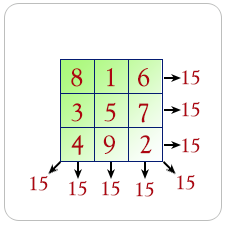# Python Example – Write a Python program to calculate magic square.# (Python Example for Citizen Data Scientist & Business Analyst)

Write a Python program to calculate magic square.

A magic square is an arrangement of distinct numbers (i.e., each number is used once), usually integers, in a square grid, where the numbers in each row, and in each column, and the numbers in the main and secondary diagonals, all add up to the same number, called the “magic constant.” A magic square has the same number of rows as it has columns, and in conventional math notation, “n” stands for the number of rows (and columns) it has. Thus, a magic square always contains n2 numbers, and its size (the number of rows [and columns] it has) is described as being “of order n”.

Pictorial presentation:Sample Solution:

Python Code:

``````
def magic_square_test(my_matrix):
iSize = len(my_matrix)
sum_list = []

Horizontal Part:
sum_list.extend([sum (lines) for lines in my_matrix])

Vertical Part:
for col in range(iSize):
sum_list.append(sum(row[col] for row in my_matrix))

Diagonals Part
result1 = 0
for i in range(0,iSize):
result1 +=my_matrix[i][i]
sum_list.append(result1)

result2 = 0
for i in range(iSize-1,-1,-1):
result2 +=my_matrix[i][i]
sum_list.append(result2)

if len(set(sum_list))>1:
return False
return True

m=[[7, 12, 1, 14], [2, 13, 8, 11], [16, 3, 10, 5], [9, 6, 15, 4]]
print(magic_square_test(m));

m=[[2, 7, 6], [9, 5, 1], [4, 3, 8]]
print(magic_square_test(m));

m=[[2, 7, 6], [9, 5, 1], [4, 3, 7]]
print(magic_square_test(m));
``````

Sample Output:

```True
True
False```

## Write a Python program to calculate magic square

#### Free Machine Learning & Data Science Coding Tutorials in Python & R for Beginners. Subscribe @ Western Australian Center for Applied Machine Learning & Data Science.```